# 关于中国农村居民收入影响因素的计量报告 Measurement Report on Influencing Factors of Rural Residents’ Income in China

• 全文下载: PDF(3554KB)    PP.678-693   DOI: 10.12677/SA.2018.76078
• 下载量: 252  浏览量: 529

China is a big agricultural country. For thousands of years, the traditional primitive and backward farming society has made the development of agriculture lag behind the development of the whole social economy
. The key to China’s development in the new century is to solve the problem of peasants’ development. Its essence is to increase the actual income of peasants. In order to study the influencing factors of rural residents’ income in China, this paper establishes a model and tests it, and concludes that there is a significant linear relationship between total crop sown area and per capita gross domestic product (GDP) and rural residents' income, and there is a positive correlation in the economic perspective.

1. 引言

2. 相关理论文献与数据来源

2.1. 文献综述

2.1.1. 人均国内总产值

2.1.2. 农产品生产价格指数

2.1.3. 化肥施用量

2.1.4. 农作物总播种面积

2.2. 数据来源Table 1. Relevant years and data

3. 模型的建立

ADF检验及协协整检验

$Y={\beta }_{0}+{\beta }_{1}{X}_{1}+{\beta }_{2}{X}_{2}+{\beta }_{3}{X}_{3}+{\beta }_{4}{X}_{4}+\mu$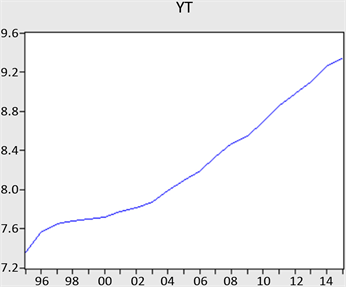Figure 1. Graphic inspection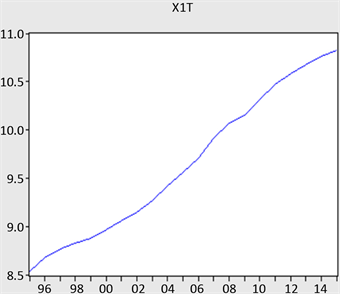Figure 2. Graphic inspection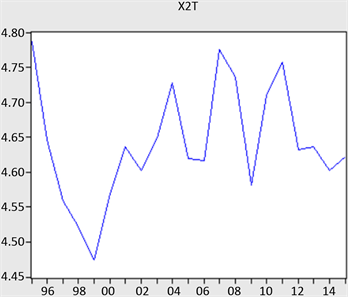Figure 3. Graphic inspection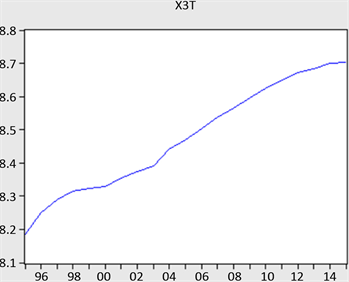Figure 4. Graphic inspection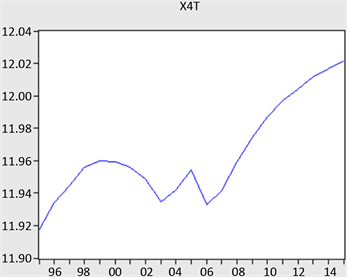Figure 5. Graphic inspection

YT：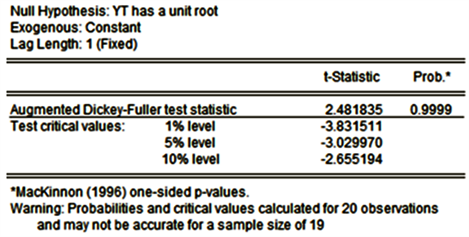Figure 6. Cointegration relationship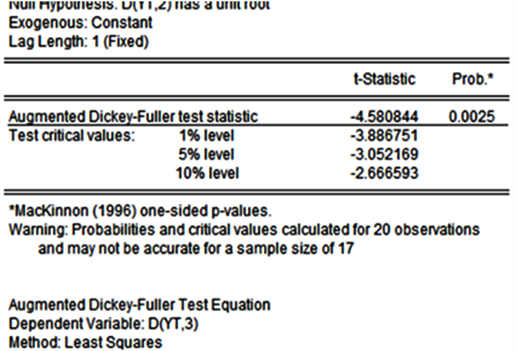Figure 7. ADF

X1T：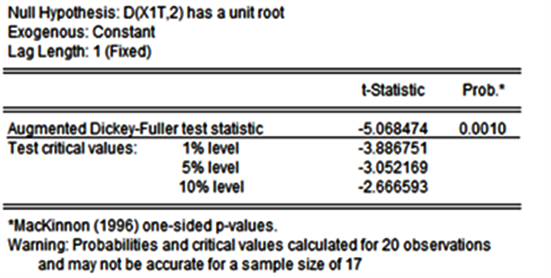Figure 8. ADF

X2T：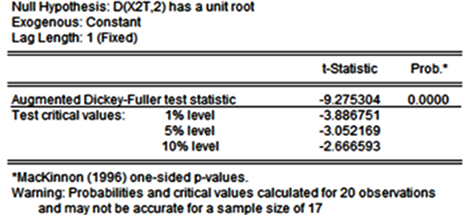Figure 9. ADF

X3T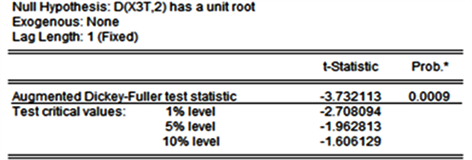Figure 10. ADF

X4T：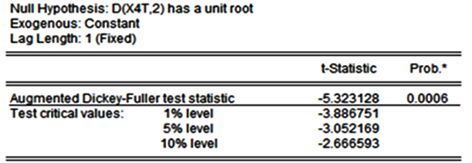Figure 11. ADF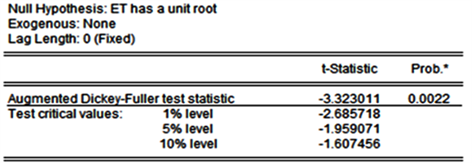Figure 12. ADF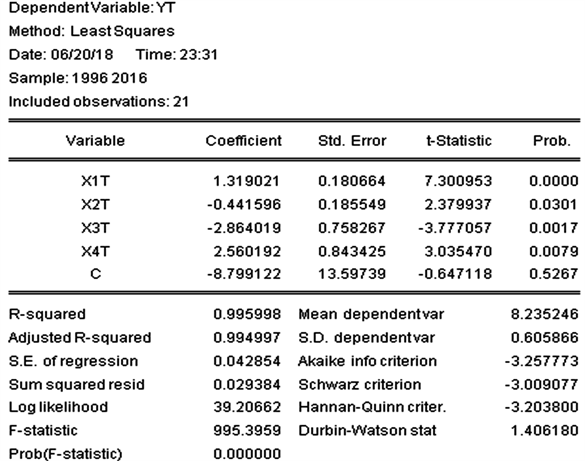Figure 13. Parameter of Model Regression Result

$\begin{array}{l}\text{YT}=-\text{8}.\text{799122}+1.319021*\text{X1T}-0.44159\text{6}*\text{X2T}-2.8640\text{19}*\text{X3T}+\text{2}.\text{56}0\text{192}*\text{X4T}\\ \text{SE}=13.59739\text{\hspace{0.17em}}\text{\hspace{0.17em}}\text{\hspace{0.17em}}\text{\hspace{0.17em}}\text{\hspace{0.17em}}\text{\hspace{0.17em}}0.180664\text{\hspace{0.17em}}\text{\hspace{0.17em}}\text{\hspace{0.17em}}\text{\hspace{0.17em}}\text{\hspace{0.17em}}\text{\hspace{0.17em}}\text{\hspace{0.17em}}\text{\hspace{0.17em}}\text{\hspace{0.17em}}\text{\hspace{0.17em}}\text{\hspace{0.17em}}0.185549\text{\hspace{0.17em}}\text{\hspace{0.17em}}\text{\hspace{0.17em}}\text{\hspace{0.17em}}\text{\hspace{0.17em}}\text{\hspace{0.17em}}\text{\hspace{0.17em}}\text{\hspace{0.17em}}\text{\hspace{0.17em}}\text{\hspace{0.17em}}\text{\hspace{0.17em}}\text{\hspace{0.17em}}0.758267\text{\hspace{0.17em}}\text{\hspace{0.17em}}\text{\hspace{0.17em}}\text{\hspace{0.17em}}\text{\hspace{0.17em}}\text{\hspace{0.17em}}\text{\hspace{0.17em}}\text{\hspace{0.17em}}\text{\hspace{0.17em}}\text{\hspace{0.17em}}\text{\hspace{0.17em}}\text{\hspace{0.17em}}0.843425\\ \text{T}=\left(-0.\text{647118}\right)\text{\hspace{0.17em}}\text{\hspace{0.17em}}\text{\hspace{0.17em}}\text{\hspace{0.17em}}\left(\text{7}.\text{3}00\text{953}\right)\text{\hspace{0.17em}}\text{\hspace{0.17em}}\text{\hspace{0.17em}}\text{\hspace{0.17em}}\text{\hspace{0.17em}}\text{\hspace{0.17em}}\text{\hspace{0.17em}}\text{\hspace{0.17em}}\left(-\text{2}.\text{379937}\right)\text{\hspace{0.17em}}\text{\hspace{0.17em}}\text{\hspace{0.17em}}\text{\hspace{0.17em}}\text{\hspace{0.17em}}\text{\hspace{0.17em}}\text{\hspace{0.17em}}\left(-\text{3}.\text{777}0\text{57}\right)\text{\hspace{0.17em}}\text{\hspace{0.17em}}\text{\hspace{0.17em}}\text{\hspace{0.17em}}\text{\hspace{0.17em}}\text{\hspace{0.17em}}\text{\hspace{0.17em}}\text{\hspace{0.17em}}\left(\text{3}.0\text{3547}0\right)\\ {R}^{2}=0.995998\text{\hspace{0.17em}}\text{\hspace{0.17em}}\text{\hspace{0.17em}}\text{\hspace{0.17em}}\text{\hspace{0.17em}}\text{\hspace{0.17em}}\text{\hspace{0.17em}}\text{\hspace{0.17em}}\text{\hspace{0.17em}}\stackrel{¯}{{R}^{2}}=0.994997\text{\hspace{0.17em}}\text{\hspace{0.17em}}\text{\hspace{0.17em}}\text{\hspace{0.17em}}\text{\hspace{0.17em}}\text{\hspace{0.17em}}\text{\hspace{0.17em}}\text{\hspace{0.17em}}F=995.3959\text{\hspace{0.17em}}\text{\hspace{0.17em}}\text{\hspace{0.17em}}\text{\hspace{0.17em}}\text{\hspace{0.17em}}\text{\hspace{0.17em}}\text{\hspace{0.17em}}\text{\hspace{0.17em}}\text{\hspace{0.17em}}\text{\hspace{0.17em}}\text{D}.\text{W}.=\text{1}.\text{4}0\text{618}\end{array}$

4. 模型检验

4.1. 经济意义检验

4.2. 统计检验

4.2.1. 拟合优度检验

4.2.2. t检验

t检验中三个解释变量的t值分别是

${t}_{1}=7.300953$ ${t}_{2}=-2.379937$ ${t}_{3}=-3.777057$ ${t}_{4}=3.035470$

4.2.3. F检验

4.3. 多重共线性的检验及补救

4.3.1. 检验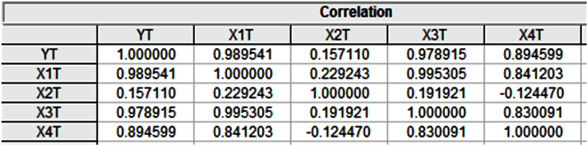Figure 14. Figure of Bivariate Coefficients of Variables

4.3.2. 分别作YT与X1T，X2T，X3T，X4T间的回归：Figure 15. Regression of YT and X1T

$\begin{array}{l}\text{YT}=0.\text{669276}+0.\text{784356}*\text{X1T}\\ \text{SE}=0.\text{253794}\text{\hspace{0.17em}}\text{\hspace{0.17em}}\text{\hspace{0.17em}}\text{\hspace{0.17em}}0.0\text{26232}\\ \text{T}=\left(\text{2}.\text{637}0\text{87}\right)\text{\hspace{0.17em}}\text{\hspace{0.17em}}\text{\hspace{0.17em}}\left(\text{29}.\text{9}00\text{52}\right)\\ {R}^{2}=0.97919\text{\hspace{0.17em}}\text{\hspace{0.17em}}\text{\hspace{0.17em}}\text{\hspace{0.17em}}\text{D}.\text{W}.=0.197011\end{array}$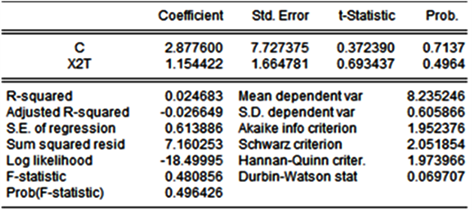Figure 16. Regression of YT and X2T

$\begin{array}{l}\text{YT}=\text{2}.\text{8776}+\text{1}.\text{154422}*\text{X2T}\\ \text{SE}=\text{7}.\text{727375}\text{\hspace{0.17em}}\text{\hspace{0.17em}}\text{1}.\text{664781}\\ \text{T}=\left(0.\text{37239}\right)\text{\hspace{0.17em}}\text{\hspace{0.17em}}\text{\hspace{0.17em}}\left(0.\text{693437}\right)\\ {R}^{2}=0.0\text{24683}\text{\hspace{0.17em}}\text{\hspace{0.17em}}\text{\hspace{0.17em}}\text{\hspace{0.17em}}\text{D}.\text{W}.=0.0\text{697}0\text{7}\end{array}$Figure 17. Regression of YT and X3T

$\begin{array}{l}\text{YT}=-\text{22}.\text{51921}+\text{3}.\text{629121}*\text{X3T}\\ \text{SE}=\text{1}.\text{472534}\text{\hspace{0.17em}}\text{\hspace{0.17em}}\text{\hspace{0.17em}}\text{\hspace{0.17em}}\text{\hspace{0.17em}}\text{\hspace{0.17em}}0.\text{173733}\\ \text{T}=\left(-\text{15}.\text{29283}\right)\text{\hspace{0.17em}}\text{\hspace{0.17em}}\text{\hspace{0.17em}}\left(\text{2}0.\text{889}0\text{9}\right)\\ {R}^{2}=0.\text{958274}\text{\hspace{0.17em}}\text{\hspace{0.17em}}\text{\hspace{0.17em}}\text{\hspace{0.17em}}\text{D}.\text{W}.=0.\text{163936}\end{array}$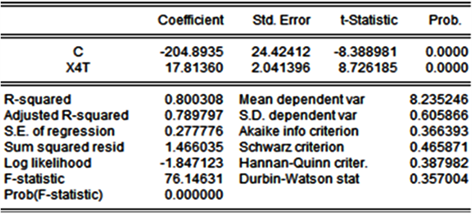Figure 18. Regression of YT and X4T

$\begin{array}{l}\text{YT}=-\text{2}0\text{4}.\text{8935}+\text{17}.\text{8136}*\text{X4T}\\ \text{SE}=\text{24}.\text{42412}\text{\hspace{0.17em}}\text{\hspace{0.17em}}\text{\hspace{0.17em}}\text{\hspace{0.17em}}\text{\hspace{0.17em}}\text{\hspace{0.17em}}\text{2}.0\text{41396}\\ \text{T}=\left(-\text{8}.\text{388981}\right)\text{\hspace{0.17em}}\text{\hspace{0.17em}}\text{\hspace{0.17em}}\left(\text{8}.\text{726185}\right)\\ {R}^{2}=0.\text{8}00\text{3}0\text{8}\text{\hspace{0.17em}}\text{\hspace{0.17em}}\text{\hspace{0.17em}}\text{\hspace{0.17em}}\text{D}.\text{W}.=0.\text{357}00\text{4}\end{array}$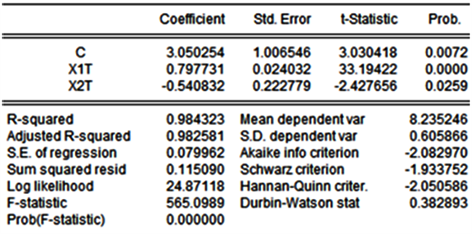Figure 19. Regression model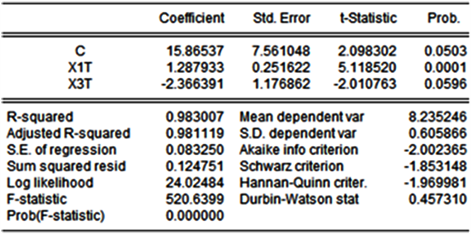Figure 20. Regression model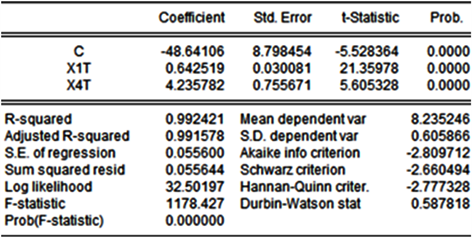Figure 21. Regression model

$\begin{array}{l}\text{Yt}=-\text{48}.\text{641}0\text{6}+0.\text{642519}*\text{X1T}+\text{4}.\text{235782}*\text{X4T}\\ \text{\hspace{0.17em}}\text{\hspace{0.17em}}\text{\hspace{0.17em}}\text{\hspace{0.17em}}\text{\hspace{0.17em}}\text{\hspace{0.17em}}\left(-\text{5}.\text{528364}\right)\text{\hspace{0.17em}}\text{\hspace{0.17em}}\text{\hspace{0.17em}}\text{\hspace{0.17em}}\left(\text{21}.\text{35978}\right)\text{\hspace{0.17em}}\text{\hspace{0.17em}}\text{\hspace{0.17em}}\text{\hspace{0.17em}}\text{\hspace{0.17em}}\text{\hspace{0.17em}}\text{\hspace{0.17em}}\text{\hspace{0.17em}}\text{\hspace{0.17em}}\left(\text{5}.\text{6}0\text{5328}\right)\end{array}$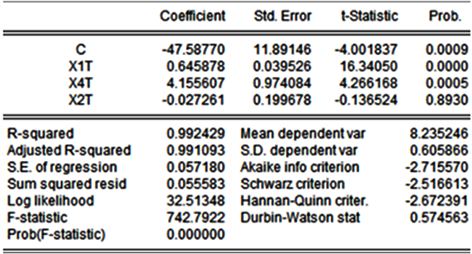Figure 22. Regression model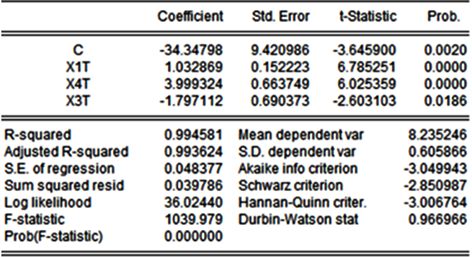Figure 23. Regression model

$\begin{array}{l}\text{YT}=-\text{48}.\text{641}0\text{6}+0.\text{642519}*\text{X1T}+\text{4}.\text{235782}*\text{X4T}\\ \text{SE}=\text{8}.\text{798454}\text{\hspace{0.17em}}\text{\hspace{0.17em}}\text{\hspace{0.17em}}\text{\hspace{0.17em}}\text{\hspace{0.17em}}\text{ }\text{ }0.0\text{3}00\text{81}\text{\hspace{0.17em}}\text{\hspace{0.17em}}\text{\hspace{0.17em}}\text{\hspace{0.17em}}\text{\hspace{0.17em}}\text{\hspace{0.17em}}\text{\hspace{0.17em}}\text{\hspace{0.17em}}\text{\hspace{0.17em}}\text{\hspace{0.17em}}\text{\hspace{0.17em}}\text{\hspace{0.17em}}0.\text{755671}\\ \text{T}=\left(-\text{5}.\text{528364}\right)\text{\hspace{0.17em}}\text{\hspace{0.17em}}\text{\hspace{0.17em}}\left(\text{21}.\text{35978}\right)\text{\hspace{0.17em}}\text{\hspace{0.17em}}\text{\hspace{0.17em}}\text{\hspace{0.17em}}\text{\hspace{0.17em}}\text{\hspace{0.17em}}\text{\hspace{0.17em}}\text{\hspace{0.17em}}\text{\hspace{0.17em}}\text{ }\text{ }\left(\text{5}.\text{6}0\text{5328}\right)\\ {R}^{2}=0.992421\text{\hspace{0.17em}}\text{\hspace{0.17em}}\text{\hspace{0.17em}}\text{\hspace{0.17em}}F=1178.427\text{\hspace{0.17em}}\text{\hspace{0.17em}}\text{\hspace{0.17em}}\text{\hspace{0.17em}}\text{DW}=0.587818\end{array}$

4.3.3. 序列相关检验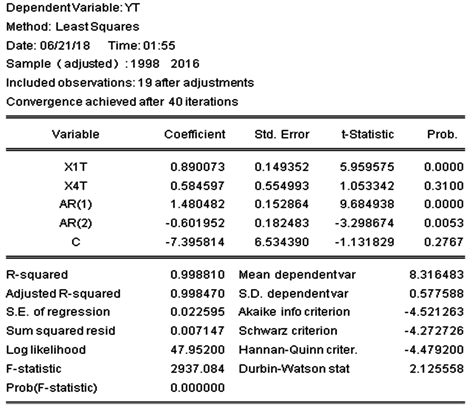Figure 24. Cochran-Ocot iteration method

DW = 2.125558，位于du < DW < 4-du之间，说明在5%显著性水平下广义差分模型中无自相关。

4.3.4. 异方差性检验

4.3.5. 协整检验修正

$\Delta Y=\alpha +{\beta }_{1}\Delta \text{X1T}+{\beta }_{4}\Delta \text{X4T}+\gamma E+\mu$

$\text{DYT}=\Delta \text{YT}=\text{YT}-\text{YT}\left(-1\right)$

$\text{DX1T}=\Delta \text{X1T}=\text{X1T}-\text{X1T}\left(-1\right)$

$\text{DX4T}=\Delta \text{X4T}=\text{X4T}-\text{X4T}\left(-1\right)$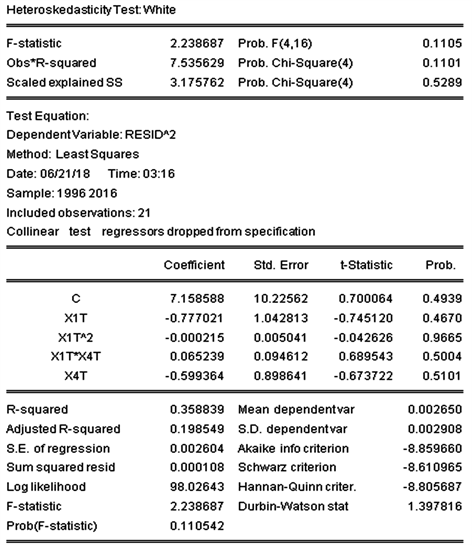Figure 25. Test result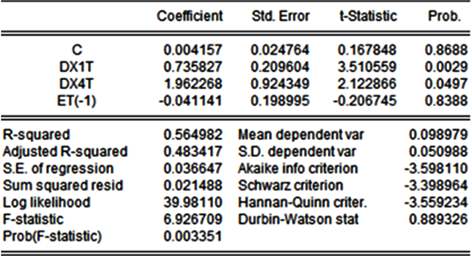Figure 26. Regression model

$\begin{array}{l}\Delta \text{YT}=0.004157+0.735827\Delta \text{X1T}+1.962268\Delta \text{X4T}-0.041141\text{ET}\\ \text{T}=0.167848\text{\hspace{0.17em}}\text{\hspace{0.17em}}\text{\hspace{0.17em}}\text{\hspace{0.17em}}\text{\hspace{0.17em}}\text{\hspace{0.17em}}\text{\hspace{0.17em}}3.510559\text{\hspace{0.17em}}\text{\hspace{0.17em}}\text{\hspace{0.17em}}\text{\hspace{0.17em}}\text{\hspace{0.17em}}\text{\hspace{0.17em}}\text{\hspace{0.17em}}\text{\hspace{0.17em}}\text{\hspace{0.17em}}\text{\hspace{0.17em}}\text{\hspace{0.17em}}\text{\hspace{0.17em}}2.122866\text{\hspace{0.17em}}\text{\hspace{0.17em}}\text{\hspace{0.17em}}\text{\hspace{0.17em}}\text{\hspace{0.17em}}\text{\hspace{0.17em}}\text{\hspace{0.17em}}\text{\hspace{0.17em}}\text{\hspace{0.17em}}\text{\hspace{0.17em}}\text{\hspace{0.17em}}-0.106745\\ {R}^{2}=0.564982\text{\hspace{0.17em}}\text{\hspace{0.17em}}\text{\hspace{0.17em}}\text{\hspace{0.17em}}\text{\hspace{0.17em}}\text{DW}=0.\text{889326}\end{array}$

4.3.6. 格兰杰因果检验

Quick/group statistics/Granger causality test，在lags to include中输入2并点击OK，得到检验结果见图27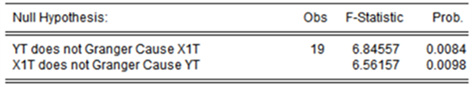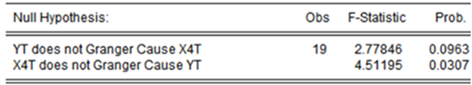Figure 27. Test result

5. 模型的确定

$\begin{array}{l}\text{YT}=-\text{48}.\text{641}0\text{6}+0.\text{642519}*\text{X1T}+\text{4}.\text{235782}*\text{X4T}\\ \text{SE}=8.798454\text{\hspace{0.17em}}\text{\hspace{0.17em}}\text{\hspace{0.17em}}\text{\hspace{0.17em}}\text{\hspace{0.17em}}\text{\hspace{0.17em}}0.030081\text{\hspace{0.17em}}\text{\hspace{0.17em}}\text{\hspace{0.17em}}\text{\hspace{0.17em}}\text{\hspace{0.17em}}\text{\hspace{0.17em}}\text{\hspace{0.17em}}\text{\hspace{0.17em}}\text{\hspace{0.17em}}\text{\hspace{0.17em}}\text{\hspace{0.17em}}\text{\hspace{0.17em}}0.755671\\ \text{T}=\left(-\text{5}.\text{528364}\right)\text{\hspace{0.17em}}\text{\hspace{0.17em}}\text{\hspace{0.17em}}\text{ }\left(\text{21}.\text{35978}\right)\text{\hspace{0.17em}}\text{\hspace{0.17em}}\text{\hspace{0.17em}}\text{\hspace{0.17em}}\text{\hspace{0.17em}}\text{\hspace{0.17em}}\text{\hspace{0.17em}}\text{\hspace{0.17em}}\text{\hspace{0.17em}}\left(\text{5}.\text{6}0\text{5328}\right)\\ {R}^{2}=0.992421\text{\hspace{0.17em}}\text{\hspace{0.17em}}\text{\hspace{0.17em}}\text{\hspace{0.17em}}\text{\hspace{0.17em}}\text{\hspace{0.17em}}F=1178.427\text{\hspace{0.17em}}\text{\hspace{0.17em}}\text{\hspace{0.17em}}\text{\hspace{0.17em}}\text{\hspace{0.17em}}\text{DW}=0.\text{587818}\end{array}$

6. 多元线性模型分析及结论

6.1. 剔除解释变量的分析

6.2. 保留解释变量分析

6.2.1. 人均国内总产值

6.2.2. 农作物总播种面积

6.2.3. 政策建议

  徐英岚. 韩村河地区农耕文化的发展现状研究[J]. 产业与科技论坛, 2013, 12(24): 31-32.  邹东涛, 栾大鹏. 完善社会主义市场经济体制的经验总结[J]. 乌鲁木齐职业大学学报, 2014, 23(1): 13-19.  赵传超, 尚珍艳. ARMA模型在成都市人均GDP预测中的应用[J]. 鲁东大学学报(自然科学版), 2012, 28(3): 223-226.  蒋燕. FDI流入对我国进出口贸易影响的实证分析[J]. 中国商贸, 2012(28): 201-202.  韩梅. 中国能源消耗影响因素的计量分析[J]. 绿色财会, 2013(7): 42-45.  郑姗, 宗义湘. 我国农业人均纯收入影响因素分析[J]. 合作经济与科技, 2015(1): 14-16.  辛永容, 肖俊哲. 我国农村居民地区间收入差异成因的实证分析[J]. 安徽商贸职业技术学院学报(社会科学版), 2008(2): 16-19.  王小平. 种植业结构调整与农村居民收入区域差异关系分析——以宜春市为例[J]. 广东农业科学, 2011, 38(16): 170-172.  胡学锋. 构建和谐社会的影响因素分析[J]. 财经理论与实践, 2005(3): 117-121.